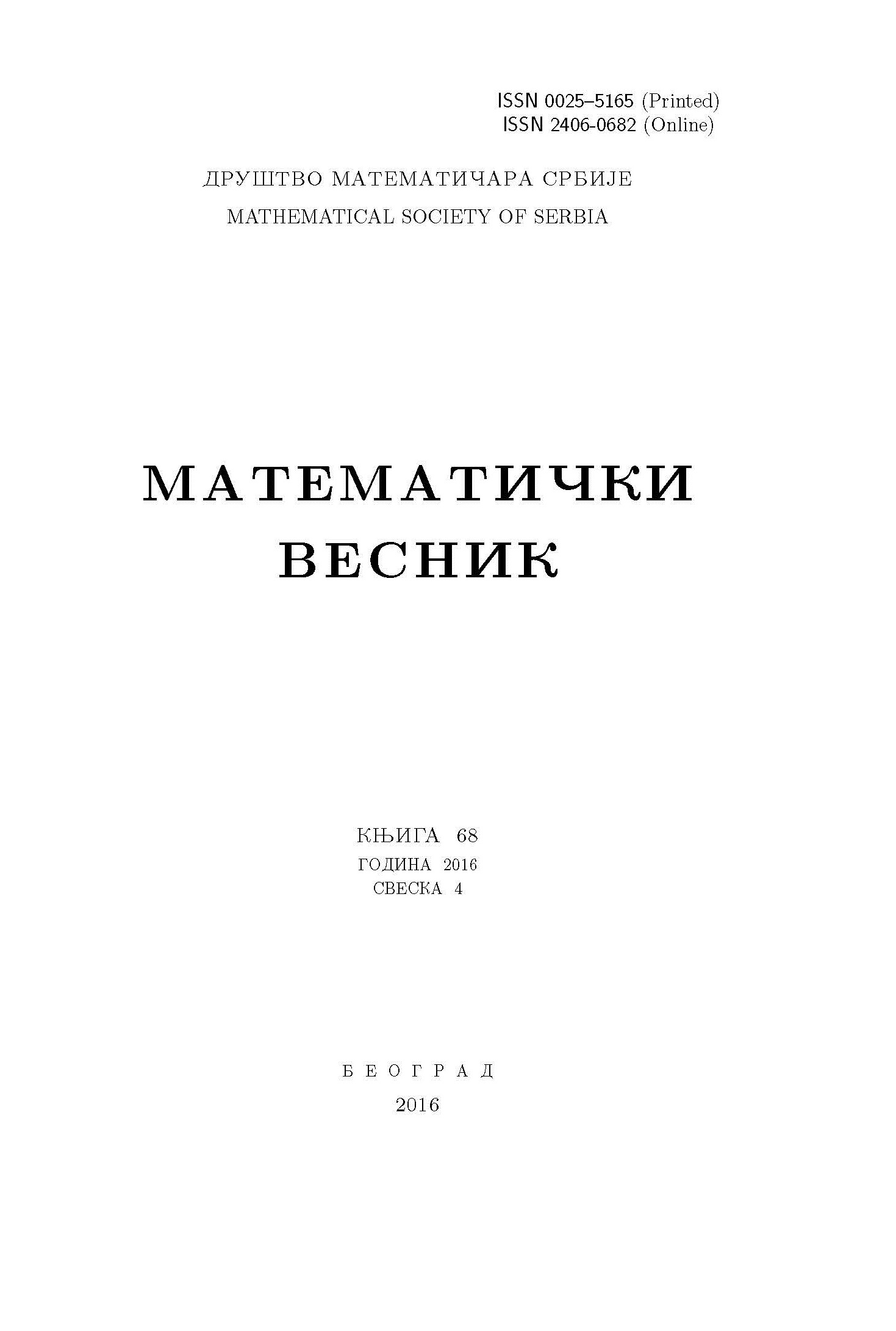﻿ Matematički Vesnik ﻿
 MATEMATIČKI VESNIK МАТЕМАТИЧКИ ВЕСНИКA GENERALIZATION OF NONSINGULAR REGULAR MAGIC SQUARES P. Jitjankarn, T. Rungratgasame AbstractA generalization of regular magic squares with magic sum $\mu$ is an sq-corner (or square corner) magic square. It is a magic square satisfying the condition that the sum of 4 entries, square symmetrically placed with respect to the center, equals $\frac{4\mu}{n}$. Using the sq-corner magic squares of order $n$, a construction of sq-corner magic squares of order {$n+2$} is derived. Moreover, this construction provides some nonsingular classical sq-corner magic squares of all orders. In particular, a nonsingular regular magic square of any odd order can be constructed under this new method, as well.Keywords: Nonsingular matrices; determinants; magic squares. MSC: 15A15, 15F10 Pages:  272$-$279 Volume  74 ,  Issue  4 ,  2022

﻿